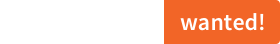# sciss / kollflitz  0.2.4Useful methods for Scala collections. Mirror of https://codeberg.org/sciss/KollFlitz

Scala versions: 3.x 2.13 2.12 2.11 2.10
Scala.js versions: 1.x

# KollFlitz

## Statement

KollFlitz provides extension methods for the Scala standard collection library. Things that I tend to require often, like calculating mean, variance or percentiles, mapping groups, counting occurrences etc., as well as a number of collection operations available in SuperCollider.

It is released under the GNU Lesser General Public License v2.1+ and comes with absolutely no warranties. To contact the author, send an email to `contact at sciss.de`.

## Note

This is used for scientific projects and rapid prototyping. The emphasis is not on performance optimisation.

## Requirements / Installation

This project builds with sbt against Scala 2.13, 2.12 (JVM), and Scala 2.13 (JS). The last version to support Scala 2.11 was v0.2.3.

To use the library in your project:

``````"de.sciss" %% "kollflitz" % v
``````

The current version `v` is `"0.2.4"`.

To play around with these operations, run `sbt console`.

## Operations

### On any type of collection, sequential or not (`Iterable`)

method description example
`counted` creates a map from elements to the frequencies of their occurrence. `List(13, 5, 8, 21, 3, 8).counted == Map(3 -> 1, 5 -> 1, 8 -> 2, 13 -> 1, 21 -> 1)`
`meanVariance` returns a tuple of mean and variance, using an implicit `Fractional` type class. `List(13.0, 5.0, 9.0).meanVariance == (9.0, 32.0)`
`mean` returns the mean value, using an implicit `Fractional` type class. `List(13.0, 5.0, 9.0).mean == 9.0`
`variance` returns the variance value, using an implicit `Fractional` type class. `List(13.0, 5.0, 9.0).variance == 5.0`
`normalized` transforms the elements by dividing them by the maximum absolute value. `List(13.0, 5.0, -9.0).normalized == List(1.0, 0.3846, -0.6923)`
`toMultiMap` takes a key and a value view function and produces a multi-map. `List("a1", "b1", "a2", "b3", "c2", "c3").toMultiMap(_.head)(_.drop(1).toInt) == Map(b -> Vector(1, 3), a -> Vector(1, 2), c -> Vector(2, 3))`
`allDistinct` determines whether all elements are unique. `List(1, 2, 3).allDistinct == true && List(1, 2, 1).allDistinct == false`

### On sequential collections (`SeqLike`)

method description example
`groupWith` groups the sequence such that within the adjacent elements of each group a predicate holds. `List(13, 5, 8, 21, 3, 8).groupWith(_ > _).toVector == Vector(List(13, 5), List(8), List(21, 3), List(8))`
`isSorted` determines whether the collection is sorted are boolean tests. `List(1, 2, 3).isSorted == true && List(1, 2, 1).isSorted == false`
`isSortedBy` determines whether the collection is sorted (using a mapping function) `List(3, 2, 1).isSortedBy(-_) == true`
`mapPairs` a mapping operation taking a function with two arguments which is applied with adjacent elements. `List(13, 5, 8, 21, 3, 8).mapPairs(_ - _) == List(8, -3, -13, 18, -5)`
`foreachPairs` iterates using a function with two arguments which is applied with adjacent elements. `foreachPairs` is to `mapPairs` what `foreach` is to `map`.
`differentiate` is a special pair map that uses a numeric type class to calculate the pairwise differences. `List(13, 5, 8, 21, 3, 8).differentiate == List(-8, 3, 13, -18, 5)`
`integrate` the opposite of `differentiate`. It aggregates a running sum. `List(13, -8, 3, 13, -18, 5).integrate == List(13, 5, 8, 21, 3, 8)`
`stutter` repeats each element of the input collection a number of times. `List(13, -8, 3).stutter(2) == List(13, 13, -8, -8, 3, 3)`
`mirror` concatenates the collection with the tail of its reverse sequence. `List(13, -8, 3).mirror == List(13, -8, 3, -8, 13)`
`decimate` drops all but one element from each n elements. `List(13, 5, 8, 21, 3, 8).decimate(2) == List(13, 8, 3)`
`wrapAt` returns an element, wrapping the index around the boundaries `List(13, 5, 8, 21).wrapAt(-1) == 21`
`foldAt` returns an element, folding (mirroring) the index within the boundaries `List(13, 5, 8, 21).foldAt(-1) == 5`
`clipAt` returns an element, clipping the index to the boundaries `List(13, 5, 8, 21).clipAt(-1) == 13`
`minIndex` determines the index of the minimum element `List(13, 5, 8, 21).minIndex == 1`
`maxIndex` determines the index of the maximum element `List(13, 5, 8, 21).maxIndex == 3`
`minIndexBy` determines the index of the minimum element, based on a mapping function `List(13, 5, 8, 21).minIndexBy(-_) == 3`
`maxIndexBy` determines the index of the maximum element, based on a mapping function `List(13, 5, 8, 21).maxIndexBy(-_) == 1`

`sortedT`, `sortByT`, `sortedWithT` are just forwarders, but they tag the result with type `Sorted` to prevent mistakes when calling methods such as `percentile` which assume that the collection is already sorted. Thus you can write `List(1, 3, 2, 7).sortedT.median` but not `List(1, 3, 2, 7).sorted.median`.

### On sorted indexed collections (`IndexedSeq @@ Sorted`)

method description example
`percentile` returns a given percentile of the sorted collection. It picks an integer index and does not interpolate
values. `Vector(13, 5, 8, 21, 3, 8).sortedT.percentile(75) == 13`
`median` a shortcut for `percentile(50)`

### Random operations

Random operation require an implicit value of type `scala.util.Random`. They must be imported via `RandomOps._`.

method description
`choose` returns a random element of a sequence.
`shuffle` returns a copy of the collection where the order of elements is randomised.
`toUrn` returns a scrambled iterator (an argument specifies whether it is infinite or not)

## Limitations

The acrobatics for allowing `String` and `Array` to be extended by these operations have not been performed.